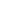Sunday , 25 September 2022

# Number Tricks – 2

####  Tell the missing digit[Step 1] : Choose a large number of six or seven digits.

[Step 2] : Take the sum of digits.

[Step 3] : Subtract sum of digits from any number chosen.

[Step 4] : Mix up the digits of resulting number.

[Step 5] : Add 25 to it.

[Step 6] : Cross out any one digit except zero.

[Step 7] : Tell the sum of the digits.

From the sum of digits you can tell the digit crossed out.

In order to find out the missing digit, subtract the sum of the digits from 25. The difference is the missing digit.

##### Example

[Step 1] : Number chosen — 1234567

[Step 2] : Sum of digits — 28

[Step 3] : 1234567-28 — 1234539

[Step 4] : Mix up the digits of resulting number. — 1235349

[Step 5] : 1235349 + 25 — 1235374

[Step 6] : Cross out digit — 5

[Step 7] : The sum of digits — 20

Number crossed out : 25-20 = 5

####  The predicted total of 1089Here is an amusing tricks with numbers. Tell your friend that you can predict his answer on a piece of paper. You have to write 1089 on a piece of paper. Let him put it in his pocket where you hardly touch it. The game begins now.

Let him write any three-digit number. It should be such that the left hand or hundred’s digit exceeds the right hand or unit’s digit by two or beyond.

Suppose he assumes 981. Tell him to write it down backwards underneath his first number. That would make it 189. Tell him to subtract the smaller one from the larger. It will be 792.

He had to add to the result the same number reversed i.e. 792 + 297. The result is 1089. The process moves as:

##### Process
 Number assumed 761 321 After reversal 167 123Subtraction 594 198 (smaller one from larger)Answer reversed 495 891 Addition 1089 1089

When your friend opens your predicted tag and sees that you have written 1089 he shall wonder how you arrived at, ahead of time.

####  To tell an integer from one digitThink a number between 1 and 9.

Annex a zero to it.

Add the following number :

Multiply this sum by 3, 11 and 3 successively.

Ask the last digit of the answer.

From this you can tell complete number as follows:

##### Process
• You know the last digit.
• For second digit, subtract the last digit from 9.
• The first digit will be one more than the second digit.
• This is the first digit of the original number.
• The third digit will be the first digit subtracted from 9.
• Arrange this and the complete number is with you.
##### Example

[Step 1] : Number thought — 3

[Step 2] : Annexing zero — 30

[Step 3] : Adding the number — 33

[Step 4] : Multiplying by 3 — 99

[Step 5] : Multiplying by 11 — 1089

[Step 6] : Multiplying by 3 — 3267

⇒ Last digit — 7

⇒ Second digit (9-7) — 2

⇒ First digit (2+1) — 3

⇒ Third digit (9-3) — 6

## साप्ताहिक टैरो राशिफल अक्टूबर 2022

साप्ताहिक टैरो राशिफल: टैरो रीडिंग (Tarot Reading in Hindi) एक प्राचीन भविष्यसूचक प्रणाली है जिसका …